Properties of Logarithms and Exponents

Laws of Logarithms

1.2.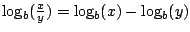3.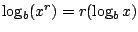Laws of Exponents

1.2.3.Ex 1 Simplify the expression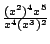.

Sol.

Ex 2 Simplify the expression.

Sol.

Pr A Find all solutions of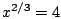.

Pr B Simplify the expression.

Pr C Use properties of logarithms to rewrite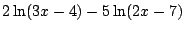as the logarithm of a single expression.

Pr 1 Simplify the expression.

Pr 2 Simplify the expression.

Pr 3 If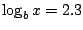and, find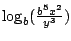.

Pr 4 Use properties of logarithms to rewriteas the logarithm of a single expression.

Pr 5 Simplify the expression.

Pr 6 Simplify the expression.

Pr 7 Simplify the expression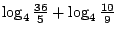.

Pr 8 Ifand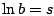, find.

Lawrence Marx 2002-07-13